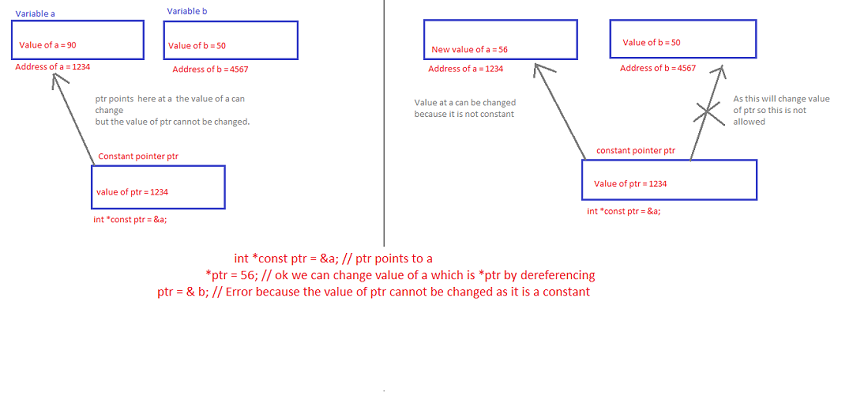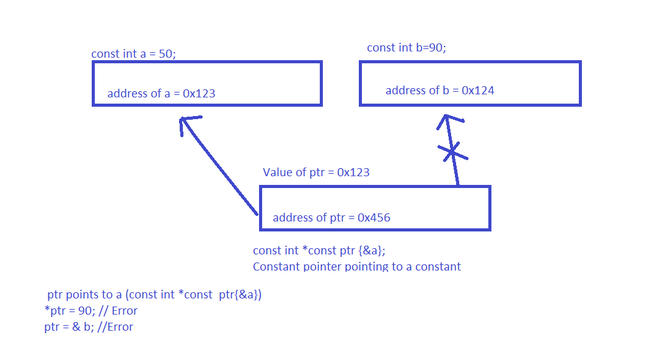Open in App
Not now

# Difference between constant pointer, pointers to constant, and constant pointers to constants

• Difficulty Level : Medium
• Last Updated : 17 Oct, 2022

In this article, we will discuss the differences between constant pointer, pointers to constant & constant pointers to constants. Pointers are the variables that hold the address of some other variables, constants, or functions. There are several ways to qualify pointers using const.

• Pointers to constant.
• Constant pointers.
• Constant pointers to constant.

Pointers to constant:

In the pointers to constant, the data pointed by the pointer is constant and cannot be changed. Although, the pointer itself can change and points somewhere else (as the pointer itself is a variable).

Below is the program to illustrate the same:

s

## C++

 `// C++ program to illustrate concept` `// of the pointers to constant` `#include ` `using` `namespace` `std;`   `// Driver Code` `int` `main()` `{`   `    ``int` `high{ 100 };` `    ``int` `low{ 66 };` `    ``const` `int``* score{ &high };`   `    ``// Pointer variables are read from` `    ``// the right to left` `    ``cout << *score << ``"\n"``;`   `    ``// Score is a pointer to integer` `    ``// which is constant *score = 78`   `    ``// It will give you an Error:` `    ``// assignment of read-only location` `    ``// ‘* score’ because value stored in` `    ``// constant cannot be changed` `    ``score = &low;`   `    ``// This can be done here as we are` `    ``// changing the location where the` `    ``// score points now it points to low` `    ``cout << *score << ``"\n"``;`   `    ``return` `0;` `}`

Output:

```100
66```

Constant pointers:

In constant pointers, the pointer points to a fixed memory location, and the value at that location can be changed because it is a variable, but the pointer will always point to the same location because it is made constant here.

Below is an example to understand the constant pointers with respect to references. It can be assumed references as constant pointers which are automatically dereferenced. The value passed in the actual parameter can be changed but the reference points to the same variable.Below is the program to illustrate the same:

## C++

 `// C++ program to illustrate concept` `// of the constant pointers` `#include ` `using` `namespace` `std;`   `// Driver Code` `int` `main()` `{`   `    ``int` `a{ 90 };` `    ``int` `b{ 50 };`   `    ``int``* ``const` `ptr{ &a };` `    ``cout << *ptr << ``"\n"``;` `    ``cout << ptr << ``"\n"``;`   `    ``// Address what it points to`   `    ``*ptr = 56;`   `    ``// Acceptable to change the` `    ``// value of a`   `    ``// Error: assignment of read-only` `    ``// variable ‘ptr’` `    ``// ptr = &b;`   `    ``cout << *ptr << ``"\n"``;` `    ``cout << ptr << ``"\n"``;`   `    ``return` `0;` `}`

Output:

```90
0x7ffc641845a8
56
0x7ffc641845a8```

Constant Pointers to constants:

In the constant pointers to constants, the data pointed to by the pointer is constant and cannot be changed. The pointer itself is constant and cannot change and point somewhere else. Below is the image to illustrate the same:Below is the program to illustrate the same:

## C++

 `// C++ program to illustrate concept of` `// the constant pointers to constant` `#include ` `using` `namespace` `std;`   `// Driver Code` `int` `main()` `{`   `    ``const` `int` `a{ 50 };` `    ``const` `int` `b{ 90 };`   `    ``// ptr points to a` `    ``const` `int``* ``const` `ptr{ &a };`   `    ``// *ptr = 90;` `    ``// Error: assignment of read-only` `    ``// location ‘*(const int*)ptr’`   `    ``// ptr = &b;` `    ``// Error: assignment of read-only` `    ``// variable ‘ptr’`   `    ``// Address of a` `    ``cout << ptr << ``"\n"``;`   `    ``// Value of a` `    ``cout << *ptr << ``"\n"``;`   `    ``return` `0;` `}`

Output:

```0x7ffea7e22d68
50```

My Personal Notes arrow_drop_up
Related Articles